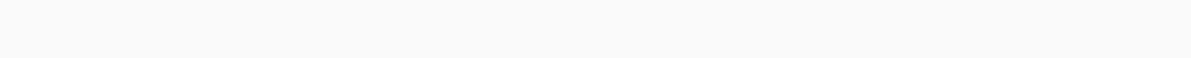# Method sympy.combsimp() in Python

Hello everyone, in this tutorial, we will learn how to implement method sympy.combsimp() and its use in Python. Please go through this tutorial carefully for better understanding and further implementation of the sympy module and the function sympy.combsimp(). The concepts used are quite easy to learn.

## Method sympy.combsimp()

This method is useful for evaluating and simplifying combinatorial expressions and therefore sympy stands for symbolic mathematics.

Combinatorial Expression:  An expression that contains more than one expression of the same type is known as a combinatorial expression such as factorial, binomial expressions, etc.

Eg. : exp = n!/(n-1)! + n!/(n-2)!

Hence it is generally used to simplify bigger mathematical calculations and formulas.

`Syntax: sympy.combsimp(expression)`

The sympy.combsimp()  method takes as input an expression containing binomials, factorials, and other “combinatorial” functions. It tries to minimize the number of combinatorial functions.  Hence it reduces the size of their arguments. So the result is given in terms of factorial, binomial, etc. The algorithm of this method works by returning all the combinatorial functions as expression. After returning it solves them. It has inbuilt mathematical functions that simplify and produce simplified expression.

So the method sympy.combsimp() method uses the concept of recurrence relation where every term is dependent on its previous or other terms. Hence the concept of recurrence relation helps it to simplify the expression easily and faster.### Examples

```import sympy
from sympy import combsimp
from sympy import factorial

expr = factorial(n)/factorial(n-3)
print(combsimp(expr))```
`Output: n*(n-1)*(n-2)`

Explanation:

In the above example, it simplifies the expression by solving the factorial of the numerator as well as the denominator. It simplifies the common terms and produces an expression.

factorial(n) = n*(n-1)*……..*2*1

```import sympy
from sympy import combsimp
from sympy import binomial
from sympy.abc import n,k

expr = binomial(n+2,k+1)/binomial(n,k)
print(combsimp(expr))```
`Output: (n+1)(n+2)/(k+1)`

Explanation:

In the above example, it simplifies the expression by opening the binomial expression of the numerator as well as the denominator. It simplifies the common terms and produces an expression.

binomial(t,k) =There are many approaches for this method but I have given you the best and the simple approach to implement and to use the method sympy.combsimp().

I hope you would be benefited with this tutorial.

You can also read about How to sort a Numpy array in Python – Various Ways?